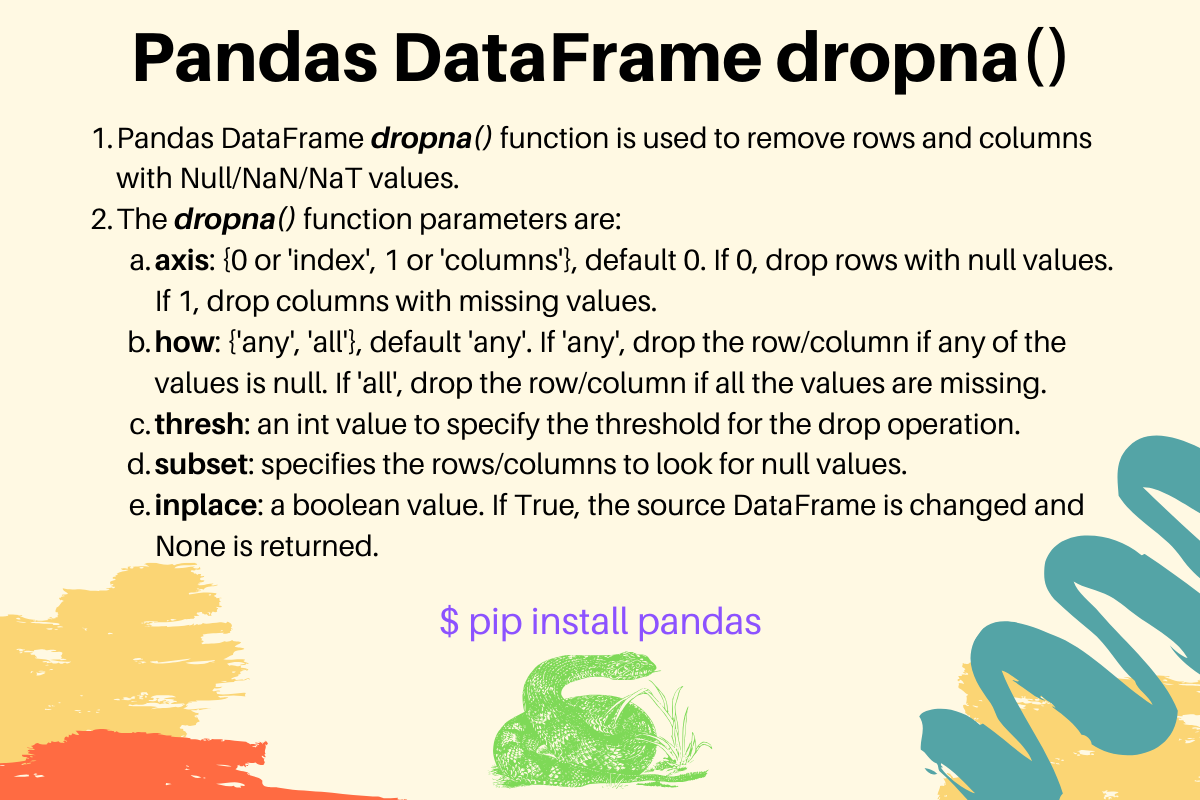# Pandas dropna() - Drop Null/NA Values from DataFrame

Published on August 3, 2022By Pankaj
Developer and author at DigitalOcean.While we believe that this content benefits our community, we have not yet thoroughly reviewed it. If you have any suggestions for improvements, please let us know by clicking the “report an issue“ button at the bottom of the tutorial.

## 1. Pandas DataFrame dropna() Function

Pandas DataFrame dropna() function is used to remove rows and columns with Null/NaN values. By default, this function returns a new DataFrame and the source DataFrame remains unchanged. We can create null values using None, pandas.NaT, and numpy.nan variables. The dropna() function syntax is:

``````dropna(self, axis=0, how="any", thresh=None, subset=None, inplace=False)
``````
• axis: possible values are {0 or ‘index’, 1 or ‘columns’}, default 0. If 0, drop rows with null values. If 1, drop columns with missing values.
• how: possible values are {‘any’, ‘all’}, default ‘any’. If ‘any’, drop the row/column if any of the values is null. If ‘all’, drop the row/column if all the values are missing.
• thresh: an int value to specify the threshold for the drop operation.
• subset: specifies the rows/columns to look for null values.
• inplace: a boolean value. If True, the source DataFrame is changed and None is returned.

Let’s look at some examples of using dropna() function.

## 2. Pandas Drop All Rows with any Null/NaN/NaT Values

This is the default behavior of dropna() function.

``````import pandas as pd
import numpy as np

d1 = {'Name': ['Pankaj', 'Meghna', 'David', 'Lisa'], 'ID': [1, 2, 3, 4], 'Salary': [100, 200, np.nan, pd.NaT],
'Role': ['CEO', None, pd.NaT, pd.NaT]}

df = pd.DataFrame(d1)

print(df)

# drop all rows with any NaN and NaT values
df1 = df.dropna()
print(df1)
``````

Output:

``````     Name  ID Salary Role
0  Pankaj   1    100  CEO
1  Meghna   2    200  None
2   David   3    NaN  NaT
3    Lisa   4    NaT  NaT

Name  ID Salary Role
0  Pankaj   1    100  CEO
``````

## 3. Drop All Columns with Any Missing Value

We can pass `axis=1` to drop columns with the missing values.

``````df1 = df.dropna(axis=1)
print(df1)
``````

Output:

``````     Name  ID
0  Pankaj   1
1  Meghna   2
2   David   3
3    Lisa   4
``````

## 4. Drop Row/Column Only if All the Values are Null

``````import pandas as pd
import numpy as np

d1 = {'Name': ['Pankaj', 'Meghna', 'David', pd.NaT], 'ID': [1, 2, 3, pd.NaT], 'Salary': [100, 200, np.nan, pd.NaT],
'Role': [np.nan, np.nan, pd.NaT, pd.NaT]}

df = pd.DataFrame(d1)

print(df)

df1 = df.dropna(how='all')
print(df1)

df1 = df.dropna(how='all', axis=1)
print(df1)
``````

Output:

``````     Name   ID Salary Role
0  Pankaj    1    100  NaT
1  Meghna    2    200  NaT
2   David    3    NaN  NaT
3     NaT  NaT    NaT  NaT

Name ID Salary Role
0  Pankaj  1    100  NaT
1  Meghna  2    200  NaT
2   David  3    NaN  NaT

Name   ID Salary
0  Pankaj    1    100
1  Meghna    2    200
2   David    3    NaN
3     NaT  NaT    NaT
``````

## 5. DataFrame Drop Rows/Columns when the threshold of null values is crossed

``````import pandas as pd
import numpy as np

d1 = {'Name': ['Pankaj', 'Meghna', 'David', pd.NaT], 'ID': [1, 2, pd.NaT, pd.NaT], 'Salary': [100, 200, np.nan, pd.NaT],
'Role': [np.nan, np.nan, pd.NaT, pd.NaT]}

df = pd.DataFrame(d1)

print(df)

df1 = df.dropna(thresh=2)
print(df1)
``````

Output:

``````     Name   ID Salary Role
0  Pankaj    1    100  NaT
1  Meghna    2    200  NaT
2   David  NaT    NaN  NaT
3     NaT  NaT    NaT  NaT

Name ID Salary Role
0  Pankaj  1    100  NaT
1  Meghna  2    200  NaT
``````

The rows with 2 or more null values are dropped.

## 6. Define Labels to look for null values

``````import pandas as pd
import numpy as np

d1 = {'Name': ['Pankaj', 'Meghna', 'David', 'Lisa'], 'ID': [1, 2, 3, pd.NaT], 'Salary': [100, 200, np.nan, pd.NaT],
'Role': ['CEO', np.nan, pd.NaT, pd.NaT]}

df = pd.DataFrame(d1)

print(df)

df1 = df.dropna(subset=['ID'])
print(df1)
``````

Output:

``````     Name   ID Salary Role
0  Pankaj    1    100  CEO
1  Meghna    2    200  NaN
2   David    3    NaN  NaT
3    Lisa  NaT    NaT  NaT

Name ID Salary Role
0  Pankaj  1    100  CEO
1  Meghna  2    200  NaN
2   David  3    NaN  NaT
``````

We can specify the index values in the subset when dropping columns from the DataFrame.

``````df1 = df.dropna(subset=[1, 2], axis=1)
print(df1)
``````

Output:

``````     Name   ID
0  Pankaj    1
1  Meghna    2
2   David    3
3    Lisa  NaT
``````

The ‘ID’ column is not dropped because the missing value is looked only in index 1 and 2.

## 7. Dropping Rows with NA inplace

We can pass `inplace=True` to change the source DataFrame itself. It’s useful when the DataFrame size is huge and we want to save some memory.

``````import pandas as pd

d1 = {'Name': ['Pankaj', 'Meghna'], 'ID': [1, 2], 'Salary': [100, pd.NaT]}

df = pd.DataFrame(d1)

print(df)

df.dropna(inplace=True)
print(df)
``````

Output:

``````     Name  ID  Salary
0  Pankaj   1   100.0
1  Meghna   2     NaN

Name  ID  Salary
0  Pankaj   1   100.0
``````

## 8. ReferencesPankaj

author

Developer and author at DigitalOcean.

#### Still looking for an answer?

Thank u bro, well explained in very simple way

- KHAJA MOINUDDIN KHAN

thats very comprehensive. out of all drop explanation … this is the best thank you

- johny